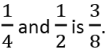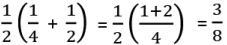Test: Number System- Assertion & Reason Type Questions

# Test: Number System- Assertion & Reason Type Questions - Class 8

Test Description

## 10 Questions MCQ Test Advance Learner Course: Mathematics (Maths) Class 8 - Test: Number System- Assertion & Reason Type Questions

Test: Number System- Assertion & Reason Type Questions for Class 8 2023 is part of Advance Learner Course: Mathematics (Maths) Class 8 preparation. The Test: Number System- Assertion & Reason Type Questions questions and answers have been prepared according to the Class 8 exam syllabus.The Test: Number System- Assertion & Reason Type Questions MCQs are made for Class 8 2023 Exam. Find important definitions, questions, notes, meanings, examples, exercises, MCQs and online tests for Test: Number System- Assertion & Reason Type Questions below.
Solutions of Test: Number System- Assertion & Reason Type Questions questions in English are available as part of our Advance Learner Course: Mathematics (Maths) Class 8 for Class 8 & Test: Number System- Assertion & Reason Type Questions solutions in Hindi for Advance Learner Course: Mathematics (Maths) Class 8 course. Download more important topics, notes, lectures and mock test series for Class 8 Exam by signing up for free. Attempt Test: Number System- Assertion & Reason Type Questions | 10 questions in 20 minutes | Mock test for Class 8 preparation | Free important questions MCQ to study Advance Learner Course: Mathematics (Maths) Class 8 for Class 8 Exam | Download free PDF with solutions
 1 Crore+ students have signed up on EduRev. Have you?
Test: Number System- Assertion & Reason Type Questions - Question 1

### DIRECTION : In the following questions, a statement of assertion (A) is followed by a statement of reason (R). Mark the correct choice as:Assertion : 5 is a rational number.Reason : The square roots of all positive integers are irrationals.

Detailed Solution for Test: Number System- Assertion & Reason Type Questions - Question 1
Here reason is not true.

√4 = ±2, which is not an irrational number.

Correct option is (c) Assertion (A) is true but reason (R) is false.

Test: Number System- Assertion & Reason Type Questions - Question 2

### DIRECTION : In the following questions, a statement of assertion (A) is followed by a statement of reason (R). Mark the correct choice as: Assertion : Sum of two irrational numbers is an irrational number. Reason : Sum of a rational number and an irrational numbers is always an irrational number.

Detailed Solution for Test: Number System- Assertion & Reason Type Questions - Question 2

Explanation:
The statement in the assertion is correct. The sum of two irrational numbers is always an irrational number. This can be proved by contradiction.

Let's assume that the sum of two irrational numbers is a rational number. In that case, we can express the sum as a fraction p/q, where p and q are integers and q is not equal to zero. Since the sum is irrational, at least one of the two numbers being added must also be irrational. Let's say one of them is irrational number a.

Now, we can rewrite the sum as a + (p/q - a). The expression p/q - a is rational because it is obtained by subtracting an irrational number from a rational number.

So, the sum a + (p/q - a) is now irrational + rational, which should be rational according to the reason given in the statement. However, we already assumed that the sum is irrational. This is a contradiction, which means that our assumption was wrong. Therefore, the sum of two irrational numbers is always irrational.

Hence, the reason provided in the statement is the correct explanation of the assertion.

Test: Number System- Assertion & Reason Type Questions - Question 3

### DIRECTION : In the following questions, a statement of assertion (A) is followed by a statement of reason (R). Mark the correct choice as:Assertion : 78 ፥ 74 = 74Reason : If a > 0 be a real number and p and q be rational numbers. Then ap x aq = ap + q.

Detailed Solution for Test: Number System- Assertion & Reason Type Questions - Question 3
We know that If a > 0 be a real number and p and q be rational numbers then ap x aq = ap+q.

So, Reason is correct.

Now, 78 ፥ 74 = 78 – 4 = 74 (∵ ap ፥ aq = ap – q) So, Assertion is also correct.

But reason (R) is not the correct explanation of assertion (A)

Correct option is (b) Both assertion (A) and reason (R) are true but reason (R) is not the correct explanation of assertion (A).

Test: Number System- Assertion & Reason Type Questions - Question 4

DIRECTION : In the following questions, a statement of assertion (A) is followed by a statement of reason (R). Mark the correct choice as:

Assertion : √5 is an irrational number.

Reason : A number is called irrational, if it cannot be written in the form p/q, where p and q are integers and q ≠ 0.

Detailed Solution for Test: Number System- Assertion & Reason Type Questions - Question 4
We know that “A number is called irrational, if it cannot be written in the form p/q, where p and q are integers and q ≠ 0.” So, Reason is correct.

Since, √5 cannot be written in the form of p/q, therefore it is an irrational number. Hence assertion is correct follows from reason.

Correct option is (a) Both assertion (A) and reason (R) are true and reason (R) is the correct explanation of assertion (A).

Test: Number System- Assertion & Reason Type Questions - Question 5

DIRECTION : In the following questions, a statement of assertion (A) is followed by a statement of reason (R). Mark the correct choice as:

Assertion : The rationalizing factor of 3 + 2√5 is 3 – 2√5.

Reason : If the product of two irrational numbers is rational then each one is called the rationalising factor of the other.

Detailed Solution for Test: Number System- Assertion & Reason Type Questions - Question 5
We know that If the product of two irrational numbers is rational then each one is called the rationalising factor of the other. So, Reason is correct.

Now, (3 + 2√5) x (3 – 2√5) = 32 – (2√5)2

= 9 – 20 = – 11

So, both Assertion and Reason are correct and Reason explains Assertion.

Correct option is (a) Both assertion (A) and reason (R) are true and reason (R) is the correct explanation of assertion (A).

Test: Number System- Assertion & Reason Type Questions - Question 6

DIRECTION : In the following questions, a statement of assertion (A) is followed by a statement of reason (R). Mark the correct choice as:

Assertion : Rational number lying between two rational numbers x and y is

½ (x + y).

Reason : There is one rational number lying between any two rational numbers.

Detailed Solution for Test: Number System- Assertion & Reason Type Questions - Question 6
We know that there are infinitely many rational numbers between any two given rational numbers.

So, Reason is not correct.

One of the rational number lying between two rational numbers x and y is ½ (x + y).

So, Assertion is correct

Correct option is (c) Assertion (A) is true but reason (R) is false.

Test: Number System- Assertion & Reason Type Questions - Question 7

DIRECTION : In the following questions, a statement of assertion (A) is followed by a statement of reason (R). Mark the correct choice as:

Assertion : Sum of two irrational numbers 2 + √3 and 4 + √3 is irrational number.

Reason : Sum of two irrational numbers is always an irrational number.

Detailed Solution for Test: Number System- Assertion & Reason Type Questions - Question 7
Here, 2 + √3 + 4 + √3 = 6 + 2√3 which is an irrational number.

So, Assertion is correct.

Now, 2 + √3 and 4 – √3 are two irrational numbers Sum = 2 + √3 + 4 – √3 = 6 which is a rational number.

So, Reason is not correct.

Correct option is (c) Assertion (A) is true but reason (R) is false.

Test: Number System- Assertion & Reason Type Questions - Question 8

DIRECTION : In the following questions, a statement of assertion (A) is followed by a statement of reason (R). Mark the correct choice as:

Assertion : 113 x 114 = 1112

Reason : If a > 0 be a real number and p and q be rational numbers.

Then ap x aq = ap + q.

Detailed Solution for Test: Number System- Assertion & Reason Type Questions - Question 8
We know that If a > 0 be a real number and p and q be rational numbers then ap x aq = ap+q.

So, Reason is true.

Now, 113 x 114 = 113+4 = 117

Here assertion is incorrect but reason is correct.

Correct option is (d) Assertion (A) is false but reason (R) is true.

Test: Number System- Assertion & Reason Type Questions - Question 9

DIRECTION : In the following questions, a statement of assertion (A) is followed by a statement of reason (R). Mark the correct choice as:

Q. Assertion : Rational number lying betweenReason : Rational number lying between two rational numbers x and y is ½(x y).

Detailed Solution for Test: Number System- Assertion & Reason Type Questions - Question 9
We know that Rational number lying between two rational numbers x and y is ½ (x + y).

So, Reason is not correct.

Now,So, Assertion is correct

Correct option is (c) Assertion (A) is true but reason (R) is false.

Test: Number System- Assertion & Reason Type Questions - Question 10

DIRECTION : In the following questions, a statement of assertion (A) is followed by a statement of reason (R). Mark the correct choice as:

Assertion : 0.329 is a terminating decimal.

Reason : A decimal in which a digit or a set of digits is repeated periodically, is called a repeating, or a recurring, decimal.

Detailed Solution for Test: Number System- Assertion & Reason Type Questions - Question 10
We know that a decimal in which a digit or a set of digits is repeated periodically, is called a repeating, or a recurring, decimal.

So, Reason is correct.

Also, we know that a decimal that ends after a finite number of digits is called a terminating decimal.

Hence Assertion is correct but reason is not the correct explanation of Assertion Correct option is (b) Both assertion (A) and reason (R) are true but reason (R) is not the correct explanation of assertion (A).

## Advance Learner Course: Mathematics (Maths) Class 8

13 videos|62 docs|24 tests
Information about Test: Number System- Assertion & Reason Type Questions Page
In this test you can find the Exam questions for Test: Number System- Assertion & Reason Type Questions solved & explained in the simplest way possible. Besides giving Questions and answers for Test: Number System- Assertion & Reason Type Questions, EduRev gives you an ample number of Online tests for practice

## Advance Learner Course: Mathematics (Maths) Class 8

13 videos|62 docs|24 tests